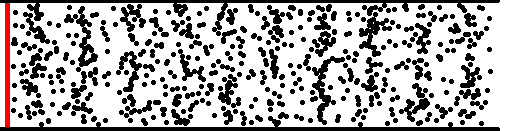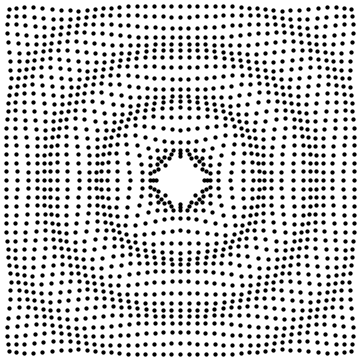﻿ 演算法筆記 - Wave

Wave (ℝ)Fourier Cosine Transform

Fourier Cosine Transform

「傅立葉餘弦轉換」是雙射函數，輸入和輸出都是一串實數，可以是離散數列或者連續函數，各有對應名稱。混淆視聽罷了。

```輸入　輸出　名稱

```Discrete Cosine Transform物理意義

N個波，頻率是0倍、0.5倍、1倍、1.5倍、……，分別是cos((2π/N)⋅0⋅t)、cos((2π/N)⋅0.5⋅t)、cos((2π/N)⋅1⋅t)、……。寫成代數是cos((2π/N)⋅(f/2)⋅t)。Discrete Cosine Transform有許多版本

N個平穩的波，微調頻率振幅相位。維基百科列出了許多版本，大家習慣以第2型做為正向轉換、以第3型做為逆向轉換。

https://en.wikipedia.org/wiki/Discrete_cosine_transform

https://en.wikipedia.org/wiki/Modified_discrete_cosine_transform

Discrete Cosine Transform數學公式

```正向餘弦轉換
N-1
y[f] =  ∑ { x[t] ⋅ cos((2π/N)⋅(f/2)⋅(t+0.5)) } ⋅ √2/N
t=0
y最後再除以√2

N-1
x[t] =  ∑ { y[f] ⋅ cos((2π/N)⋅(f/2)⋅(t+0.5)) } ⋅ √2/N
f=0
y事先要除以√2
```

Discrete Cosine Transform是線性函數Integer Discrete Cosine Transform

https://www.vcodex.com/h264avc-4x4-transform-and-quantization/

2D Discrete Cosine TransformPlot3D[Cos[1.5x]Cos[1.5y], {x, 0, 2Pi}, {y, 0, 2Pi}, PlotRange -> {-1, 1}, Axes -> False, ColorFunction -> (ColorData["CherryTones"][Rescale[#3, {-2, 2}]] &)]

Gibbs PhenomenonWave (ℂ)

Complex NumberEuler's Formulae運算簡單，考慮長度與角度即可。e性質優美，每轉90°剛好是±1與±i。也許你會漸漸愛上它。

WaveFourier Transform

Fourier Transform

「傅立葉轉換」是雙射函數，輸入輸出都是一串複數，可以是離散數列或者連續函數，各有對應名稱。混淆視聽罷了。

```輸入　輸出　名稱

```Discrete Fourier Transform物理意義

N個複數波，頻率是0倍到N-1倍，分別是ei⋅(2π/N)⋅0⋅t、ei⋅(2π/N)⋅1⋅t、……、ei⋅(2π/N)⋅(N-1)⋅t。寫成代數是ei⋅(2π/N)⋅f⋅t。（複數波很難畫，故圖例為實數波。）Discrete Fourier Transform數學公式

```正向傅立葉轉換
N-1
y[f] =  ∑ { x[t] ÷ ei⋅(2π/N)⋅f⋅t } ÷ √N
t=0
N-1
=  ∑ { x[t] ⋅ e-i⋅(2π/N)⋅f⋅t } ÷ √N
t=0

N-1
x[t] =  ∑ { y[f] ⋅ ei⋅(2π/N)⋅f⋅t } ÷ √N
f=0
```

```       N-1
y[f] =  ∑ { x[t] ÷ ei⋅(2π/N)⋅f⋅t }
t=0
N-1
x[t] =  ∑ { y[f] ⋅ ei⋅(2π/N)⋅f⋅t } ÷ N
f=0
```

Discrete Fourier Transform是線性函數

```ω = ei⋅2π/N

[y0  ]   [ ω-0⋅0     ω-0⋅1     ω-0⋅2     ..  ω-0⋅(N-1)     ]  [x0  ]
[y1  ]   [ ω-1⋅0     ω-1⋅1     ω-1⋅2     ..  ω-1⋅(N-1)     ]  [x1  ]
[y2  ] = [ ω-2⋅0     ω-2⋅1     ω-2⋅2     ..  ω-2⋅(N-1)     ]  [x2  ]
[:   ]   [ :         :         :             :            ]  [:   ]
[yN-1]   [ ω-(N-1)⋅0 ω-(N-1)⋅1 ω-(N-1)⋅2 ..  ω-(N-1)⋅(N-1) ]  [xN-1]

[x0  ]       [ ω0⋅0     ω0⋅1     ω0⋅2     ..  ω0⋅(N-1)     ]  [y0  ]
[x1  ]    1  [ ω1⋅0     ω1⋅1     ω1⋅2     ..  ω1⋅(N-1)     ]  [y1  ]
[x2  ] = ——— [ ω2⋅0     ω2⋅1     ω2⋅2     ..  ω2⋅(N-1)     ]  [y2  ]
[:   ]    N  [ :        :        :            :           ]  [:   ]
[xN-1]       [ ω(N-1)⋅0 ω(N-1)⋅1 ω(N-1)⋅2 ..  ω(N-1)⋅(N-1) ]  [yN-1]
``````                           FFT
(x₀ x₁ x₂ x₃ x₄ x₅ x₆ x₇) ----> (y₀ y₁ y₂ y₃ y₄ y₅ y₆ y₇)

N = 8, ω = e-i⋅2π/N   注意到ω放入了負號，讓下面的數學式子比較簡潔

y₀ = x₀ω⁰ + x₁ω⁰ + x₂ω⁰ + x₃ω⁰ + x₄ω⁰ + x₅ω⁰ + x₆ω⁰ + x₇ω⁰
= (x₀ω⁰ + x₂ω⁰ + x₄ω⁰ + x₆ω⁰) + (x₁ω⁰ + x₃ω⁰ + x₅ω⁰ + x₇ω⁰)
= (x₀ω⁰ + x₂ω⁰ + x₄ω⁰ + x₆ω⁰) + ω⁰ ⋅ (x₁ω⁰ + x₃ω⁰ + x₅ω⁰ + x₇ω⁰)
= (x₀ x₂ x₄ x₆)轉換結果第0項 + ω⁰ ⋅ (x₁ x₃ x₅ x₇)轉換結果第0項
= y偶0 + ω⁰ ⋅ y奇0

y₁ = x₀ω⁰ + x₁ω¹ + x₂ω² + x₃ω³ + x₄ω⁴ + x₅ω⁵ + x₆ω⁶ + x₇ω⁷
= (x₀ω⁰ + x₂ω² + x₄ω⁴ + x₆ω⁶) + (x₁ω¹ + x₃ω³ + x₅ω⁵ + x₇ω⁷)
= (x₀ω⁰ + x₂ω² + x₄ω⁴ + x₆ω⁶) + ω¹ ⋅ (x₁ω⁰ + x₃ω² + x₅ω⁴ + x₇ω⁶)
= (x₀υ⁰ + x₂υ¹ + x₄υ² + x₆υ³) + ω¹ ⋅ (x₁υ⁰ + x₃υ¹ + x₅υ² + x₇υ³)
= (x₀ x₂ x₄ x₆)轉換結果第1項 + ω¹ ⋅ (x₁ x₃ x₅ x₇)轉換結果第1項
= y偶1 + ω¹ ⋅ y奇1

y₂ = x₀ω⁰ + x₁ω² + x₂ω⁴ + x₃ω⁶ + x₄ω⁸ + x₅ω¹⁰ + x₆ω¹² + x₇ω¹⁴
= (x₀ω⁰ + x₂ω⁴ + x₄ω⁸ + x₆ω¹²) + (x₁ω² + x₃ω⁶ + x₅ω¹⁰ + x₇ω¹⁴)
= (x₀ω⁰ + x₂ω⁴ + x₄ω⁸ + x₆ω¹²) + ω² ⋅ (x₁ω⁰ + x₃ω⁴ + x₅ω⁸ + x₇ω¹²)
= (x₀υ⁰ + x₂υ² + x₄υ⁴ + x₆υ⁶ ) + ω² ⋅ (x₁υ⁰ + x₃υ² + x₅υ⁴ + x₇υ⁶ )
= (x₀ x₂ x₄ x₆)轉換結果第2項 + ω² ⋅ (x₁ x₃ x₅ x₇)轉換結果第2項
= y偶2 + ω² ⋅ y奇2

y₃ = x₀ω⁰ + x₁ω³ + x₂ω⁶ + x₃ω⁹ + x₄ω¹² + x₅ω¹⁵ + x₆ω¹⁸ + x₇ω²¹
= (x₀ω⁰ + x₂ω⁶ + x₄ω¹² + x₆ω¹⁸) + (x₁ω³ + x₃ω⁹ + x₅ω¹⁵ + x₇ω²¹)
= (x₀ω⁰ + x₂ω⁶ + x₄ω¹² + x₆ω¹⁸) + ω³ ⋅ (x₁ω⁰ + x₃ω⁶ + x₅ω¹² + x₇ω¹⁸)
= (x₀υ⁰ + x₂υ³ + x₄υ⁶  + x₆υ⁹ ) + ω³ ⋅ (x₁υ⁰ + x₃υ³  + x₅υ⁶ + x₇υ⁹ )
= (x₀ x₂ x₄ x₆)轉換結果第3項 + ω³ ⋅ (x₁ x₃ x₅ x₇)轉換結果第3項
= y偶3 + ω³ ⋅ y奇3

y₄ = x₀ω⁰ + x₁ω⁴ + x₂ω⁸ + x₃ω¹² + x₄ω¹⁶ + x₅ω²⁰ + x₆ω²⁴ + x₇ω²⁸
= (x₀ω⁰ + x₂ω⁸ + x₄ω¹⁶ + x₆ω²⁴) + (x₁ω⁴ + x₃ω¹² + x₅ω²⁰ + x₇ω²⁸)
= (x₀ω⁰ + x₂ω⁸ + x₄ω¹⁶ + x₆ω²⁴) + ω⁴ ⋅ (x₁ω⁰ + x₃ω⁸ + x₅ω¹⁶ + x₇ω²⁴)
= (x₀ω⁰ + x₂ω⁰ + x₄ω⁰  + x₆ω⁰ ) + ω⁴ ⋅ (x₁ω⁰ + x₃ω⁰ + x₅ω⁰  + x₇ω⁰ )
= (x₀ x₂ x₄ x₆)轉換結果第0項 + ω⁴ ⋅ (x₁ x₃ x₅ x₇)轉換結果第0項
= y偶0 + ω⁴ ⋅ y奇0

y₅ y₆ y₇以此類推
```
```y₀ = y偶0 + y奇0 ⋅ ω⁰
y₁ = y偶1 + y奇1 ⋅ ω¹
y₂ = y偶2 + y奇2 ⋅ ω²
y₃ = y偶3 + y奇3 ⋅ ω³
y₄ = y偶0 + y奇0 ⋅ ω⁴
y₅ = y偶1 + y奇1 ⋅ ω⁵
y₆ = y偶2 + y奇2 ⋅ ω⁶
y₇ = y偶3 + y奇3 ⋅ ω⁷
```Hartley Transform

```傅立葉轉換：
2πft           2πft    -i2πft/N
cos ———— - i ⋅ sin ———— = e
N              N

2πft           2πft       2πft
cos ———— +     sin ———— = cas ————
N              N          N

2πft           2πft
cos ———— -     sin ————
N              N
```
```傅立葉轉換：
N-1
y[f] =  ∑ { x[t] ÷ ei⋅(2π/N)⋅f⋅t } ÷ √N
t=0

N-1
y[f] =  ∑ { x[t] ⋅ cas((2π/N)⋅f⋅t) } ÷ √N
t=0
```

```  N-1
∑ { x[t] ⋅ cas((2π/N)⋅f⋅t) }
t=1,3,5,...
N/2-1
=  ∑ { x[2t+1] ⋅ cas((2π/N)⋅f⋅(2t+1)) }
t=0,1,2,...
N/2-1
=  ∑ { x[2t+1] ⋅ ( cas((2π/N)⋅f⋅2t) ⋅ cos((2π/N)⋅f⋅1)
t=0,1,2,...       + cas(-(2π/N)⋅f⋅2t) ⋅ sin((2π/N)⋅f⋅1) ) }
N/2-1
=  ∑ { x[2t+1] ⋅ ( cas((2π/(N/2))⋅f⋅t) ⋅ cos((2π/N)⋅f⋅1)
t=0,1,2,...       + cas(-(2π/(N/2))⋅f⋅t) ⋅ sin((2π/N)⋅f⋅1) ) }
N/2-1
=  ∑ { x[2t+1] ⋅ cas( (2π/(N/2))⋅f⋅t) } ⋅ cos((2π/N)⋅f⋅1)
t=0,1,2,...
N/2-1
+  ∑ { x[2t+1] ⋅ cas(-(2π/(N/2))⋅f⋅t) } ⋅ sin((2π/N)⋅f⋅1)
t=0,1,2,...

= y奇[f] ⋅ cos((2π/N)⋅f⋅1) + y奇[-f] ⋅ sin((2π/N)⋅f⋅1)
```
```θ = 2π / N
y0 = y偶0 + y奇0 * cos0θ + y奇0 ⋅ sin0θ
y1 = y偶1 + y奇1 * cos1θ + y奇3 ⋅ sin1θ
y2 = y偶2 + y奇2 * cos2θ + y奇2 ⋅ sin2θ
y3 = y偶3 + y奇3 * cos3θ + y奇1 ⋅ sin3θ
y4 = y偶0 + y奇0 * cos4θ + y奇0 ⋅ sin4θ
y5 = y偶1 + y奇1 * cos5θ + y奇3 ⋅ sin5θ
y6 = y偶2 + y奇2 * cos6θ + y奇2 ⋅ sin6θ
y7 = y偶3 + y奇3 * cos7θ + y奇1 ⋅ sin7θ
```

http://mathworld.wolfram.com/HartleyTransform.html

2D Discrete Fourier TransformFourier Transform的性質

Frequency SpectrumWindow FunctionWindow Function的快速演算法F = FourierTransform[HannWindow[x], x, w] Plot[F, {w, 0, +70}, PlotRange->{-0.05,+0.2}, Axes->None] F = FourierTransform[HannWindow[x], x, w] Plot[F, {w, 0, +70}, PlotRange->{-0.001,+0.001}, Axes->None] F = Abs[FourierTransform[HannWindow[x], x, w]] LogPlot[F, {w, 0, +70}, Axes->None]Sparse Fourier Transform

http://groups.csail.mit.edu/netmit/sFFT/

http://people.csail.mit.edu/indyk/fourier-gsip.pdf

ListPlot[Table[Sin[x*2*Pi/16], {x, 0, 15}]] ListPlot[Abs[Fourier[Table[Sin[x*2*Pi/16], {x, 0, 15}]]], PlotRange->{0, 2}, Filling->Axis] ListPlot[Arg[Fourier[Table[Sin[x*2*1.5*Pi/16], {x, 0, 15}]]], PlotRange->{-4, +4}, Filling->Axis] ListPlot[Abs[Fourier[Table[HannWindow[(x-16)/32], {x, 0, 31}]]], PlotRange->{0, 2}, Filling->Axis] ListPlot[Arg[Fourier[Table[HannWindow[(x-16)/32], {x, 0, 31}]]], PlotRange->{-4, 4}, Filling->Axis] ListPlot[Table[HannWindow[(x-16)/32], {x, 0, 31}], PlotRange->{0, 1}, Filling->Axis, FillingStyle->Red, PlotStyle->Red, Axes->None] ListPlot[Table[Cos[x*2*Pi/32] * HannWindow[(x-16)/32], {x, 0, 31}]] ListLinePlot[Table[Cos[x*2*Pi/64], {x, 0, 63}]] ListLinePlot[Abs[Fourier[Table[Cos[x*2*Pi/60], {x, 0, 63}]]], PlotRange->{0, 8}] ListLinePlot[Abs[Fourier[Table[Cos[x*2*Pi/60] * HannWindow[(x-32)/64], {x, 0, 63}]]], PlotRange->{0, 8}]

Fourier Transform的性質```加法-加法         a + b = aft + bft

```

Laplace Transform

Wavelet（Under Construction!）

Wavelet

「小波」，自訂特殊造型的波。

http://www.faculty.jacobs-university.de/llinsen/teaching/320491/Lecture05.pdf

Wavelet Transform

「小波轉換」是雙射函數，輸入和輸出都是一串實數，可以是離散數列或者連續函數。

https://en.wikipedia.org/wiki/Fast_wavelet_transform